# C++ Program to Capitalize the First Letter of Every Word in a File

This article provides a program in C++ that helps in capitalizing all the words (or every word) in a file. The file's name must be entered by the user at run-time. Capitalizing every word means converting the first letter of every word to uppercase.

#### What Should You Do Before the Program?

The program is based on the file. That is, the program given below capitalizes each and every word in a file. Therefore, we need a file (with some content) that must be available inside the current directory. Therefore, I'm going to create a file called "codescracker.txt" first.

I've created the file; here's a snapshot of the current directory (the folder where the C++ source code is saved) that contains my newly created "codescracker.txt" file: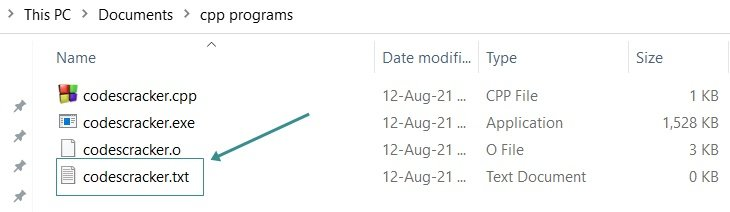Now put some content inside this file. Since the program given below capitalizes each word in a file, I'm recommending that you write all the words in small letters so that our program can be tested well. Here is a snapshot of the opened file after writing some words inside it: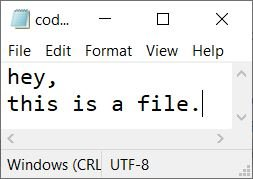Now let's move on and create a program in C++ that capitalizes all the words available in this file.

## Capitalizing all words in a file

The question is: write a program in C++ that receives the name of a file from the user at run-time and capitalizes each and every word available in that file. Here is its answer:

```#include<iostream>
#include<fstream>
#include<sstream>

using namespace std;
int main()
{
ifstream inf;
ofstream ouf;
stringstream str_stream;
string str;
char filename;
int i=0, temp=0;

cout<<"Enter File's Name: ";
cin>>filename;

inf.open(filename);
str_stream<<inf.rdbuf();
inf.close();
str = str_stream.str();
while(str[i])
{
if(temp==0)
{
str[i] = toupper(str[i]);
temp = 1;
}
else if(isspace(str[i]))
temp = 0;
i++;
}
ouf.open(filename);
ouf<<str;
cout<<"\nAll words capitalized Successfully!";
cout<<endl;
return 0;
}```

Here is the initial output produced by the above C++ program on capitalizing each word in a file given by the user: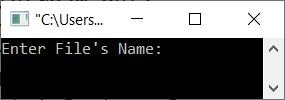Now, enter the name of the file that was created earlier in this article, "codescracker.txt", and press the ENTER key to capitalize all of the words in this file, as shown in the screenshot below: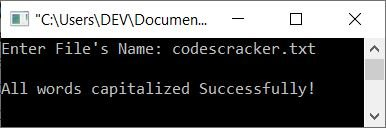Here is the new snapshot of the same file after executing the above program: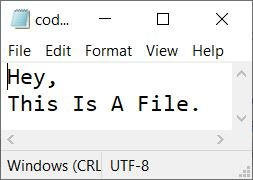As you can see from the above snapshot of the file codescracker.txt, all its words get capitalized after executing the C++ program.

Note: The stringstream class (defined in the sstream header file) allows a string object to be treated as a stream.

Note: The rdbuf() function is used to return a pointer to the stream buffer object that is currently associated with the stream.

To implement capitalization code manually (or to capitalize every word without using toupper()), you can get the code from capitalize every word in a string and utilize it in the above program. Do it yourself for your own practice.

If you are having trouble implementing the manual version of the code that capitalizes every word, then just replace the following block of code (from the above program):

```   while(str[i])
{
if(temp==0)
{
str[i] = toupper(str[i]);
temp = 1;
}
else if(isspace(str[i]))
temp = 0;
i++;
}```

with the block of code given below:

```   while(str[i])
{
if(temp==0)
{
asc_val = str[i];
if(asc_val>=97 && asc_val<=122)
{
asc_val = asc_val-32;
str[i] = asc_val;
}
temp = 1;
}
if(isspace(str[i]))
temp = 0;
i++;
}```

You will get the same output as the previous program.

C++ Quiz

« Previous Program Next Program »